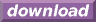#Calcute: freeware calculator

## Hyperbolic Arcsecant (hyperbolic arc-secant, hyperbolic inverse secant, asech)

The hyperbolic arc-secant is the inverse of the hyperbolic secant function. Calcute implements it with the asech function. The inverse hyperbolic secant of a value x is the value y for which the hyperbolic secant of y is x. In other words if y = asech(x) then x = sech(y).

```asech(1)
0
```

The hyperbolic arc-secant function is only defined in the semi-open range (0, 1] that matches the output domain of the hyperbolic secant function: it is undefined at 0 but defined at 1. The hyperbolic secant function is symmetric about the Y axis therefore the inverse hyperbolic secant is bi-valued: any parameter in the valid range matches both a positive and a negative number. But the asech function generates a single (principal) value: the positive one. The other, negative possibility is obtained by negating the return value of asech.

In some mathematical texts and hand-held calculators, the notation sech-1 is sometimes used to represent the hyperbolic arc-secant function. This is an unfortunate notational choice since it could also stand for the multiplicative inverse 1/sech, which is a different function.

This function is sometimes called the "area hyperbolic secant". Another abbreviation sometimes used to represent it is "arcsech".